# 3D Shapes

Here we will learn about 3D shapes, including their names and properties, surface area and volume, and plans and elevations.

There are also 3D shapes worksheets based on Edexcel, AQA and OCR exam questions which are printable, along with further guidance on where to go next if you’re still stuck.

## What are 3D shapes?

3D shapes are solid shapes that have 3 dimensions: height, width and depth

We can categorise and solve problems involving 3D shapes using a variety of methods.

### What are 3D shapes?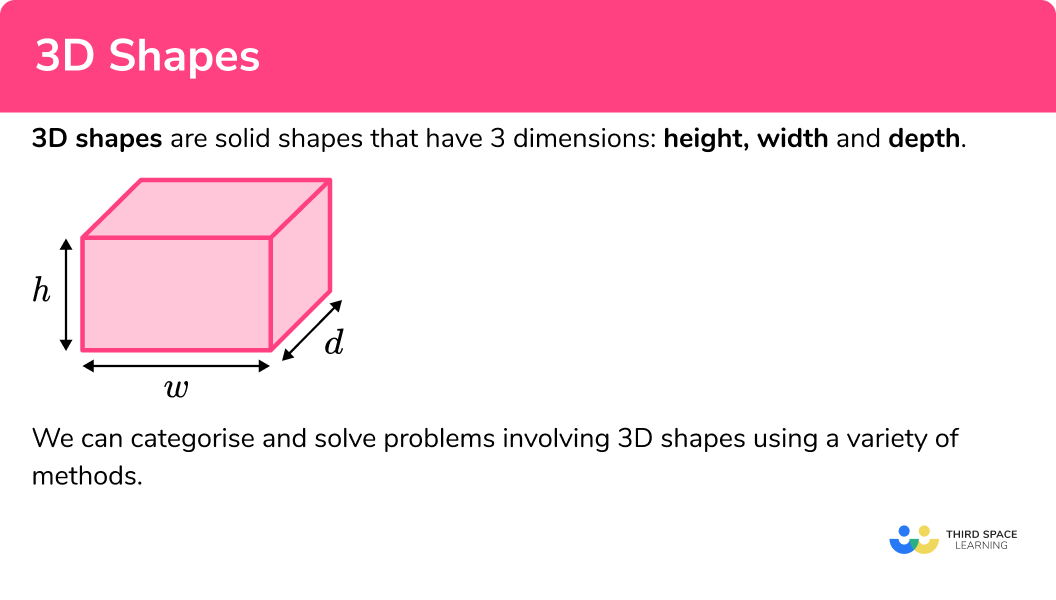### 3D shape names

3D shapes can be categorised in several ways.

Firstly a 3D shape is either a polyhedra (a 3D shape with flat polygonal faces), or a non-polyhedra (a 3D shape which has a curved surface). 3D shapes can then be further categorised as shown in the table below. The properties of 3D shapes can help to classify them and recall their names.

Note that some 3D shapes fit into more than one category.

*There are other categories of 3D shapes, but these cover what is needed for the GCSE course.

Here are some examples of common 3D shapes;

Step-by-step guide: 3D shape names

### Faces, edges and vertices

Polyhedra are 3D shapes made up of faces, edges and vertices as illustrated below.

The shape in the table is a cube. It has 6 faces, 12 edges and 8 vertices.

Step-by-step guide: Faces, edges and vertices

### Volume

The volume of a 3D shape is the amount of space there is inside the shape.

Step-by-step guide: How to calculate volume

### Surface area

Surface area is the total area occupied by the surface of a 3D shape.

To find the surface area of a prism, cylinder or pyramid it is often helpful to draw the net of the 3D shape, calculate the area of each face, and then add them together.

To find the surface area of 3D shapes with curved surfaces such as spheres and cones (higher GCSE only), there are special formulas you should use

Surface area of a sphere =4 \times \pi \times radius^{2}

Curved surface area of a cone =\pi \times radius \times length

Step-by-step guide: How to calculate surface area

### Plans and elevations

We can use isometric paper to draw 3D shapes.

For example, this 3cm \times 3cm \times 3cm cube has been drawn using the dots as guides.

We can also draw 2D images of 3D shapes from different perspectives. These are called plans and elevations.

A plan is a drawing of what a 3D shape looks like from above looking down (like the bird’s eye view). An elevation is a drawing of what a  3D shape looks like  from the side or front.

For example,

Draw the plan and side elevation of this 3D shape.

We can also recreate a 3D shape when we are given the plan and elevations

For example,

Draw the 3D shape which has the following plan and elevations.

Step-by-step guide: Plans and elevations

### Prisms

A prism is a polyhedra that has congruent cross sections. In non-mathematical words, it is a 3D shape that can be sliced like a loaf of bread, and each slice will look like the same flat shape.

For example, a triangular prism has triangular cross sections.

The net of a prism consists of two identical 2D shapes and a number of rectangles.

The number of rectangles is equal to the number of sides in the 2D shape. For example, the net of a triangular prism has 3 rectangles because a triangle has 3 sides. Below are the nets of 3 different prisms.

We can calculate the volume and surface area of prisms.

Step-by-step guide: Prism

### Cuboids

A cuboid is a polyhedron with 6 rectangular faces.

Cubes are a special type of cuboid where the length, width and height are all equal.

The net of a cuboid consists of 6 rectangles.

We can calculate the volume and surface area of cuboids.

Step-by-step guide: Cuboid

### Triangular prisms

A triangular prism is a polyhedron consisting of two triangular ends connected by three rectangles. The triangular ends of a triangular prism are congruent (exactly the same).

The net of a triangular prism consists of 3 rectangles and 2 triangles.

We can calculate the volume and surface area of triangular prisms.

Step-by-step guide: Triangular prism

### Spheres and hemispheres

A sphere is a 3D shape which can be described simply as a ball. Mathematically it is a non-polyhedra with no vertices and just a single curved surface. Every point on the surface of a sphere is equidistant from the centre. This distance is the radius of the sphere.

A hemisphere is half a sphere. It has one curved surface and one flat circular surface.

We can calculate the volume and surface area of spheres and hemispheres.

Step-by-step guide: Sphere

Step-by-step guide: Hemisphere

### Cones

Cones are like pyramids but with circular bases. The cross-sections of a cone are similar circles.

We can calculate the volume and surface area of a cone.

Step-by-step guide: Cone

### Cylinders

Geometrically a cylinder is like a prism. The cross sections of cylinders are congruent circles.

The net of a cylinder consists of two identical circles and one rectangle that folds around the circles to create a curved surface.

We can calculate the volume and surface area of a cylinder.

Step-by-step guide: Cylinder

### Pyramids

A pyramid is a 3D shape which has a flat base shape and an apex.

A pyramid has similar cross-sections as shown in this diagram.

We can calculate the volume and surface area of a pyramid.

Step-by-step guide: Pyramid

### Volume and surface area of cuboids and prisms

We can work out the volume and surface area of 3D shapes.

The volume of a 3D shape is the space inside it.

The surface area of a 3D shape is the total area of all its faces.

• Cuboids

To find the volume of a cuboid we use the formula,

\text{Volume} = \text{length} \times \text{width} \times \text{height}.

For example,

Calculate the volume of the cuboid.

\text{Volume} = \text{length} \times \text{width} \times \text{height}.

Length = 6 \ cm

Width = 2 \ cm

Height = 3 \ cm

Volume = 6 \times 2 \times 3 = 36 \ cm^3

The surface area of a cuboid can be calculated by adding together the areas of the six faces. The properties of a cuboid are such that the opposite faces are congruent rectangles.

Therefore we only need to find the area of three different rectangular faces, add them together, and then double this answer to find the total surface area.

For example,

Calculate the surface area of the cuboid.

Area front = 6 \times 4 = 24 \ cm^2

Area top = 4 \times 3 = 12 \ cm^2

Area side = 6 \times 3 = 18 \ cm^2

Total surface area

\begin{aligned} &= (24 + 12 + 18) \times 2 \\\\ &= 54 \times 2 \\\\ &= 108 \ cm^2 \end{aligned}

• Prisms

To calculate the volume of a prism, we calculate the area of the cross-section and then multiply this  by the depth.

\text{Volume of a prism } = \text{ area of cross-section } \times \text{ depth }

For example,

Calculate the volume of the prism.

This cross-section is a triangle.

Area triangle = \frac{1}{2}(\text{base} \times \text{height})

Area of the cross-section,

\begin{aligned} &= \frac{1}{2}(6 \times 4) \\\\ &= 12 \ cm^2 \end{aligned}

\text{Volume} = \text{area of cross-section} \times \text{length}

\text{Volume} = 12 \times 8 = 96 \ cm^3

The surface area of a prism can be calculated by adding together the areas of all its faces.

For example,

Calculate the surface area of the prism.

This is a triangular prism. It has 5 faces.

Area of each triangular face = \frac{1}{2} (4 \times 3) = 6 \ cm^2

Area of base rectangle = 6 \times 4 = 24 \ cm^2

Area of vertical side rectangle = 3 \times 6 = 18 \ cm^2

Area of sloping side rectangle = 6 \times 5 = 30 \ cm^2

Total surface area = 6 + 6 + 24 + 18 + 30 = 84 \ cm^2

### Volume of cylinders, pyramids, cones and spheres

• Cylinders

Geometrically a cylinder is like a prism, so we can calculate the volume using the same formula.

\text{Volume of a prism } = \text{ area of cross-section } \times \text{ depth}

The cross-section of a cylinder is a circle and so we need to remember the formula for area.

\text { Area of a circle }=\pi r^{2}

For example, calculate the volume of the cylinder.

\text{Volume } = \text{ area of cross-section } \times \text{ depth}

\begin{aligned} \text{ Volume }&= \pi r^{2} \times \text{ depth} \\\\ &=\pi \times 7^{2} \times 12 \\\\ &= 588 \pi \\\\ &= 1847.26 \ cm^3 \ (2dp) \end{aligned}

(Note that ‘height’ and ‘depth’ can be used interchangeably here.)

For example, calculate the volume of the cylinder.

\text{Volume } = \text{ area of cross-section } \times \text{ depth}

\begin{aligned} \text{Volume }&= \pi r^{2} \times \text{ depth} \\\\ &= \pi \times 4^{2} \times 10 \\\\ &= 160 \pi \\\\ & =502.65 \ cm^3 \ (2dp) \end{aligned}

• Pyramids

We can calculate the volume of a pyramid using this formula.

\text{Volume of a pyramid }=\frac{1}{3} \times \text{ area of base } \times \text{ perpendicular height}

For example, calculate the volume of the pyramid.

\text{Volume of a pyramid }=\frac{1}{3} \times \text{ area of base } \times \text{ perpendicular height}

Area of the base =6 \times 6 = 36 \ cm^2

\begin{aligned} \text{Volume } &= \frac{1}{3} \times 36 \times 4 \\\\ &= 48 \ cm^3 \end{aligned}

• Cones

We can calculate the volume of a cone using this formula.

\text{Volume}=\frac{1}{3} \pi r^2 h

For example, calculate the volume of the cone.

\begin{aligned} \text { Volume } &=\frac{1}{3} \pi r^{2} h \\\\ &=\frac{1}{3} \times \pi \times 5^{2} \times 7 \\\\ &=183.3 \mathrm{~cm}^{3}(1 d. p) \end{aligned}

• Spheres

We can calculate the volume of a sphere using the formula.

Volume = \frac{4}{3} \pi r^{3}

For example, calculate the volume of the sphere.

\begin{aligned} \text { Volume } &=\frac{4}{3} \pi r^{3} \\\\ &=\frac{4}{3} \times \pi \times 3^{2} \\\\ &=37.7 \mathrm{~cm}^{3} \end{aligned}

## How to use 3D shapes

3D shapes is a broad topic which covers many different facts and skills.

• 3D shape names
• Faces, edges and vertices
• Volume
• Surface area
• Plans and elevations
• Prisms
• Cuboids
• Triangular prisms
• Spheres
• Hemispheres
• Cones
• Cylinders
• Pyramids

### Explain how to use 3D Shapes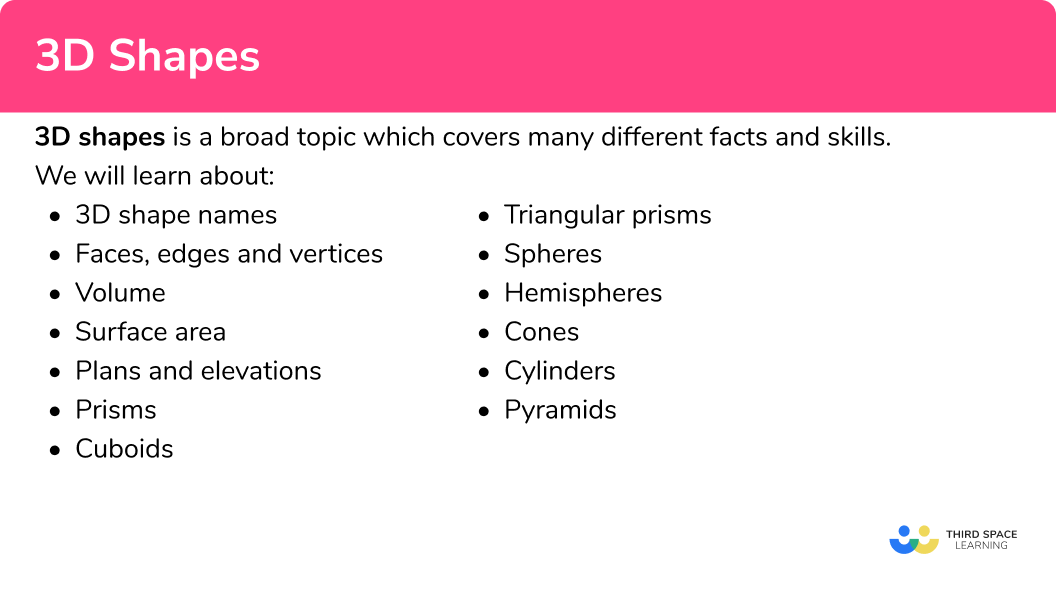## 3D shapes examples

### Example 1: 3D shape names

What is the name of this 3D shape?

1. Identify if the 3D shape is,
i)  a polyhedron (all flat polygonal faces) – go to step 2.
ii) a non-polyhedron (includes a curved surface) – go to step 3.

This 3D shape has a curved surface; go to step 3.

2Identify if all the faces are the same regular shape.
i) If yes, this is one of the platonic solids (tetrahedron, cube, octahedron, dodecahedron or icosahedron).
ii) If no, continue to step 3.

Skip this step.

3Identify if the 3D shape is
a) a pyramid or cone (3D shape with a base, an apex and similar cross-sectional areas).
b) a prism or cylinder (3D shape with congruent cross-sectional areas).
c) neither.

This 3D shape has a base, an apex and similar cross sectional areas; it is a pyramid.

4Name the shape.
a) For pyramids: the name of the base shape often forms the name of the 3D shape.
b) For prisms: the name of the cross-sectional area often forms the name of the 3D shape.
c) i) For neither (polyhedron): this is an irregular polyhedron or a compound solid.
ii) For neither (non-polyhedron): those needed to be named on the GCSE syllabus are spheres and hemispheres.

### Example 2: faces, edges and vertices

How many faces, vertices and edges does this 3D shape have?

This 3D shape is a prism. Below is a sketch of the net.

This 3D shape has 6 faces.

There are 4 edges going around the front polygon, there are 4 edges going around the back polygon, there are 4 edges joining the front and back polygons.

This gives a total of 12 edges.

There are 4 vertices at the bottom of the 3D shape. There are 4 vertices at the top of the 3D shape. This gives a total of 8 vertices.

Answer: 6 faces, 12 edges, 8 vertices.

### Example 3: volume of a sphere

Calculate the volume of a sphere with radius r=4.5cm. Write your answer correct to 2 decimal places.

The volume of a sphere

V=\frac{4}{3}\pi{r^{3}}.

Substituting r=4.5cm, into V, we have

V=\frac{4}{3}\times\pi\times{4.5^{3}}.

V=\frac{4}{3}\times\pi\times{4.5^{3}}

V=381.70351

The question asks for the answer to be correct to two decimal places.

The unit of length in the question is cm, so the answer for the volume will be in cm^{3}.

V = 381.70cm^{3}

### Example 4: plans and elevations

On square paper draw the plan, front elevation and side elevation of this 3D shape.

The plan view is taken looking down onto the shape. We should only be able to see blue squares.

The plan view consists of 3 blue squares horizontally in line with each other.

The front elevation is taken looking from the left of the shape (as labelled on the diagram). We should only be able to see pink squares.

The front elevation consists of the three rows of 1, 2, and 3 pink squares in line with each other.

The side elevation is taken looking from the right of the shape. We should only be able to see green squares.

The side elevation consists of 3 green squares vertically in line with each other.

### Example 5: surface area

Calculate the total surface area of the cylinder below. Write your answer in terms of \pi.

The net of the cylinder consists of two circles and a rectangle where the width of the rectangle is the circumference of the circle, and the height of the rectangle is the height of the prism.

The circumference of the circle is C=2\pi{r}=2\times\pi\times{3}=6\pi .

Sketching and labelling the net of the cylinder, we have

The area of the top and bottom of the cylinder are the same, moreover, they are two circles with a radius of 3cm . Using the formula for the area of a circle ( A=\pi{r}^{2} ) where r = 3 , we have

A=\pi\times{3}^{2}=\pi\times{9}=9\pi .

As there are two circles, 9\pi\times{2}=18\pi .

Important note: We are leaving the working in terms of π as the answer is also in terms of π .

The remaining rectangular face has an area of

\text{area of a rectangle }=\text{height }\times\text{width }=5\times6\pi=30\pi .

18\pi+30\pi=48\pi

The units of length in the question are in centimetres and so the units of area in the solution are in square centimetres ( cm^{2} ).

The surface area of the cylinder in terms of π is 48 \pi cm^{2} .

### Example 6: volume

Calculate the volume of the triangular prism below.

The volume of a triangular prism is

V=\text{area of the triangular cross section }\times\text{length}.

The area of a triangle is A=\frac{b\times{h}}{2} .

As b=10cm and h=13cm , substituting these into A we get

A=\frac{10\times{13}}{2}=65 .

We now have A=65cm^{2} and l=16cm which we can substitute into the formula for the volume,

V=\text{area of the triangular cross section}\times\text{length}=65\times{16} .

\begin{aligned} V&=65\times{16}\\ &=1040\\ \end{aligned}

The units in the question are in centimetres and so the solution is in cubic centimetres ( cm^{3} ).

V = 1040cm^{3}.

### Common misconceptions

• Using a descriptive 3D shape name instead of the correct mathematical name

Some 3D shapes have descriptive names or can be likened to certain categories of 3D shapes but must be referred to by the correct mathematical name. Here is a list of examples which are part of the GCSE syllabus.

Descriptive name/Incorrect name = correct mathematical name

Circular prism = cylinder

Rectangular prism = cuboid

Circular based pyramid = cone

Triangular based pyramid (equilateral triangles) = tetrahedron

Ball = sphere

Half a ball = hemisphere

• Using the wrong formula

There are several formulas that are given on the formula sheet for surface area and volume. Make sure you select the right one by correctly identifying the 3D shape in the question and what it is asking you to calculate.

• Using a mixture of units in a calculation

When you are calculating volume or surface area you must ensure that the units of length you are using are the same. This may mean that you need to convert a unit before you carry out a calculation. For example, if one length is given in metres and another is given in centimetres, you must convert one of them so that they are either both in metres or both in centimetres.

• Missing or incorrect units

Surface area is measured in units squared (e.g. mm^{2}, \ cm^{2}, \ m^{2} etc).

Volume is measured in units cubed (e.g. mm^{3}, \ cm^{3}, \ m^{3} etc).

For volume you may also be asked to give your answer in litres or millilitres for example. Note that

1 \ ml= 1 \ cm^{3}

1 \ litre = 1000 \ ml = 1000 \ cm^{3}

• Hidden faces, edges and vertices

When counting the faces, edges or vertices on an image of a 3D shape you should consider carefully if there are any that are, in a sense, ‘hidden’. Some 3D drawings use dashed lines to represent edges that cannot be seen but sometimes they do not. In this case try and visualise if there are any ‘hidden’ faces, edges or vertices behind or underneath the 3D representation.

• Rounding at multiple stages during long calculations

It is important not to round decimal answers until the end of a calculation. Rounding at multiple stages will result in an inaccurate final answer. It is a good idea to learn how to use the ‘ANS’ button or memory function on your calculator which will help you to substitute long decimal answers into subsequent calculations.

• Mixing up the front and side elevations

The front elevation and the side elevation can be labelled in different ways. The front elevation is often indicated by an arrow, like in the picture below. Always look for an indication like this and do not make your own assumption about which perspective is the front.

### Practice 3D shapes questions

1. What is the name of this 3D shape?Pentagonal PyramidPentagonal PrismPentagonIrregular PrismThis 3D shape has congruent cross-sectional areas, it is therefore a prism. The shape of the cross-section is a regular pentagon, hence, it is a pentagonal prism.

2. This is a square based pyramid. How many vertices does it have?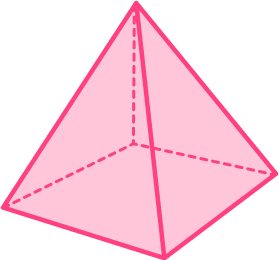4853The base of the pyramid has 4 vertices as they are the corners of a square. The apex (the highest point if the shape) is another vertex, totaling 5.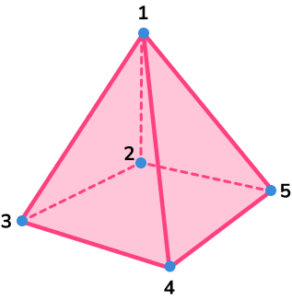3. Calculate the volume of a hemisphere with radius 5cm. Write your answer in terms of \pi .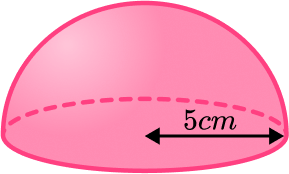262 \pi\frac{500\pi}{3}83\pi\frac{250\pi}{3}The volume of a sphere is V=\frac{4}{3}\pi r^{3}.

A hemisphere is half this value.

\begin{aligned} &V=\frac{4}{3}\pi\times{5^3}=\frac{4}{3}\pi\times{125}=\frac{500}{3}\pi \\\\ &\frac{500}{3}\pi\div{2}=\frac{250\pi}{3} \end{aligned}

4. Which of the following represents the plan view of the 3D shape below: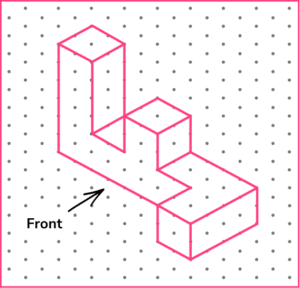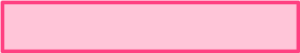The plan view is from above looking down, the bird’s eye view.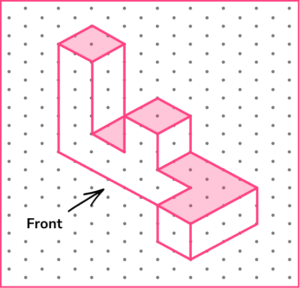5. Calculate the surface area of the triangular prism below.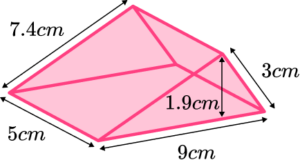114.1 \ cm^2105.55 \ cm^2999 \ cm^2131.2 \ cm^2Find the area of each face and then add these values together.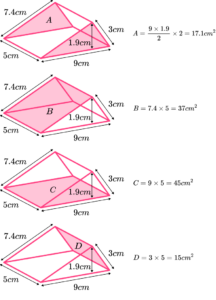17.1+37+45+15=114.1 \ cm^{2}

6. Calculate the volume of the cuboid below.181.72 \ cm^2181.72 \ cm^3150.328 \ cm^2150.328 \ cm^3The volume of a cuboid is equal to V = h \times w \times d.

Here, V = 3.8 \times 9.2 \times 4.3 = 150.328 \ cm^{3} (remember cube units for volume).

### 3D shapes GCSE questions

1. Match the solid shape to the correct mathematical name.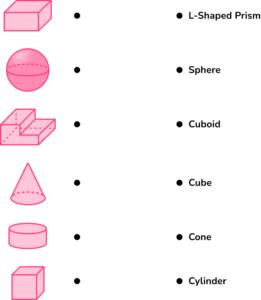(3 marks)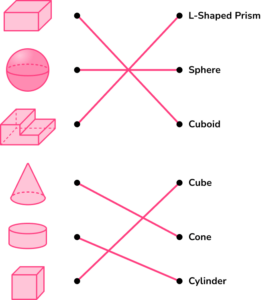For every two correct matches.

(1)

2. (a) Determine which shape has the greater volume?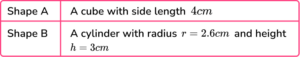(b) State the volume to surface area ratio of Shape A in its simplest form.

(6 marks)

(a)

Volume of Shape A = 4^{3} = 64 \ cm^{3}

(1)

Volume of Shape B = \pi \times r^{2} \times h = \pi \times 2.6^{2} \times 3 = 63.7 \ cm^{3}

(1)

Shape A has a greater volume.

(1)

(b)

SA = 6 \times 4^{2} = 96 \ cm^{2}

(1)

V:SA=64:96

(1)

=2:3

(1)

3. The sun is almost spherical. The diameter of the sun is approximately equal to 1.4\times 10^{6} \ km.

(a) Calculate the radius of the sun in standard form.

(b) Given that the volume of a sphere is equal to V=\frac{4}{3}\pi r^{3}, estimate the volume of the sun.

(5 marks)

(a)

1.4\times 10^{6} \div 2 = 0.7\times 10^{6}

(1)

7\times 10^{5} \ km

(1)

(b)

V=\frac{4}{3}\times\pi\times(7\times{10^{5}})^3

(1)

V=1.43675504 \times 10^{18}

(1)

V=1.4\times 10^{18} \ km^{3}

(1)

## Learning checklist

You have now learned how to:

• Identify 3D shapes, including cubes and other cuboids, from 2D representations
• Construct and interpret plans and elevations of 3D shapes
• Calculate surface areas and volumes of spheres, pyramids, cones and composite solids
• Use the properties of faces, surfaces, edges and vertices of cubes, cuboids, prisms, cylinders, pyramids, cones and spheres to solve problems in 3D
• Recognise when it is possible to use formulae for area and volume of shapes
• Compare and classify geometric shapes based on their properties and sizes

## Still stuck?

Prepare your KS4 students for maths GCSEs success with Third Space Learning. Weekly online one to one GCSE maths revision lessons delivered by expert maths tutors.

Find out more about our GCSE maths tuition programme.